## Matrix

The Transformation given by the system of equations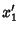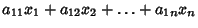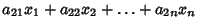is denoted by the Matrix Equation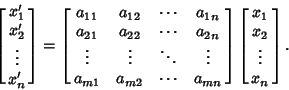In concise notation, this could be writtenwhereandare Vectors and A is called anmatrix. A matrix is said to be Square if. Special types of Square Matrices include the Identity Matrix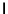, with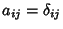(where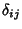is the Kronecker Delta) and the Diagonal Matrix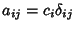(where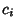are a set of constants).

For every linear transformation there exists one and only one corresponding matrix. Conversely, every matrix corresponds to a unique linear transformation. The matrix is an important concept in mathematics, and was first formulated by Sylvesterand Cayley.Two matrices may be added (Matrix Addition) or multiplied (Matrix Multiplication) together to yield a new matrix. Other common operations on a single matrix are diagonalization, inversion (Matrix Inverse), and transposition (Matrix Transpose). The Determinantorof a matrix A is a very important quantity which appears in many diverse applications. Matrices provide a concise notation which is extremely useful in a wide range of problems involving linear equations (e.g., Least Squares Fitting).

See also Adjacency Matrix, Adjugate Matrix, Antisymmetric Matrix, Block Matrix, Cartan Matrix, Circulant Matrix, Condition Number, Cramer's Rule, Determinant, Diagonal Matrix, Dirac Matrices, Eigenvector, Elementary Matrix, Equivalent Matrix, Fourier Matrix, Gram Matrix, Hilbert Matrix, Hypermatrix, Identity Matrix, Incidence Matrix, Irreducible Matrix, Kac Matrix, LU Decomposition, Markov Matrix, Matrix Addition, Matrix Decomposition Theorem, Matrix Inverse, Matrix Multiplication, McCoy's Theorem, Minimal Matrix, Normal Matrix, Pauli Matrices, Permutation Matrix, Positive Definite Matrix, Random Matrix, Rational Canonical Form, Reducible Matrix, Roth's Removal Rule, Shear Matrix, Skew Symmetric Matrix, Smith Normal Form, Sparse Matrix, Special Matrix, Square Matrix, Stochastic Matrix, Submatrix, Symmetric Matrix, Tournament Matrix

References

Arfken, G. Matrices.'' §4.2 in Mathematical Methods for Physicists, 3rd ed. Orlando, FL: Academic Press, pp. 176-191, 1985.